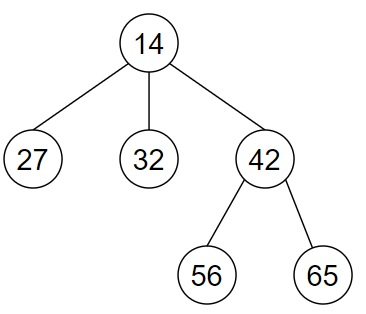# Program to make a copy of a n-ary tree in Python

Suppose, we have been provided a n-ary tree whose root is given to us 'root'. We have to make a copy of the full n-ary binary tree and perform a preorder traversal of both trees. The copied tree has to be stored using another root node. The node structure of the tree is given below −

Node:
value : <integer>
children : <array>

So, if the input is like, then the output will be

[14, 27, 32, 42, 56, 65]

The preorder representation of the input tree and the output tree will be the same as an exact copy of the tree has been created.

To solve this, we will follow these steps −

• if root is not empty, then

• return root

• head := a new node with the value of root

• q := a new deque containing elements root and head

• while q is not empty, do

• node := pop the first element from the q

• cloned := pop the first element from the q

• for each chld in node.children, do

• new_n := a new node containing the value of chld

• append new_n to the children of cloned

• insert chld and new_n at the end of q

## Example (Python)

Let us see the following implementation to get better understanding −

Live Demo

from queue import deque
class Node:
def __init__(self, value, child = None) -> None:
self.val = value
self.children = []
if child != None:
for value in child:
self.children.append(value)

def solve(root):
if not root:
return root
while q:
node, cloned = q.popleft()
for chld in node.children:
new_n = Node(chld.val)
cloned.children.append(new_n)
q.append((chld,new_n))

def treeprint(node, tree):
if node == None:
tree.append("None")
return tree
if tree == None:
tree = []
tree.append(node.val)
for child in node.children:
treeprint(child, tree)
return tree

node6 = Node(65)
node5 = Node(56)
node4 = Node(42, [node5, node6])
node3 = Node(32)
node2 = Node(27)
node1 = Node(14, [node2, node3, node4])
root = node1

copynode = solve(root)
print(treeprint(copynode, None))

## Input

node6 = Node(65)
node5 = Node(56)
node4 = Node(42, [node5, node6])
node3 = Node(32)
node2 = Node(27)
node1 = Node(14, [node2, node3, node4])
root = node1

## Output

[14, 27, 32, 42, 56, 65]